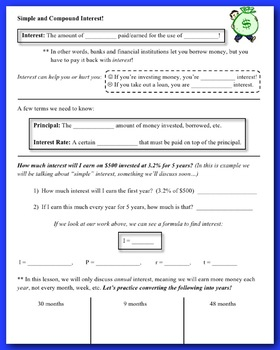# Simple and Compound Interest - Guided Notes and HomeworkSubject
Resource Type
Format
Zip (3 MB|9 pages)
Standards
\$2.00
\$2.00

### Description

This 2-day lesson includes 6 pages of guided notes, a 1-page assignment, and a 2-page assignment.

This lesson works well in both pre-algebra and algebra classes. The goal for part 1 is to understand the process of simple and compound interest, so students will use more of a step-by-step approach. But in part 2 they discover formulas and why we need them.

Students learn about simple and compound interest in the order below. They will:

- Learn important vocabulary

- Solve simple interest problems in two steps (finding the interest amount and then adding it to the original)

- Learn the difference between simple and compound interest

- Solve compound interest problems with a year-by-year approach

- On day 2, discover and use the annual compound interest formula

- Discover and use the annual simple interest formula

* Part 2 works well as an intro into linear vs. exponential relationships

Total Pages
9 pages
Included
Teaching Duration
2 days
Report this Resource to TpT
Reported resources will be reviewed by our team. Report this resource to let us know if this resource violates TpT’s content guidelines.

### Standards

to see state-specific standards (only available in the US).
Observe using graphs and tables that a quantity increasing exponentially eventually exceeds a quantity increasing linearly, quadratically, or (more generally) as a polynomial function.
Construct linear and exponential functions, including arithmetic and geometric sequences, given a graph, a description of a relationship, or two input-output pairs (include reading these from a table).
Prove that linear functions grow by equal differences over equal intervals, and that exponential functions grow by equal factors over equal intervals.
Distinguish between situations that can be modeled with linear functions and with exponential functions.
Use the properties of exponents to interpret expressions for exponential functions. For example, identify percent rate of change in functions such as y = (1.02) to the 𝘵 power, 𝘺 = (0.97) to the 𝘵 power, 𝘺 = (1.01) to the 12𝘵 power, 𝘺 = (1.2) to the 𝘵/10 power, and classify them as representing exponential growth or decay.